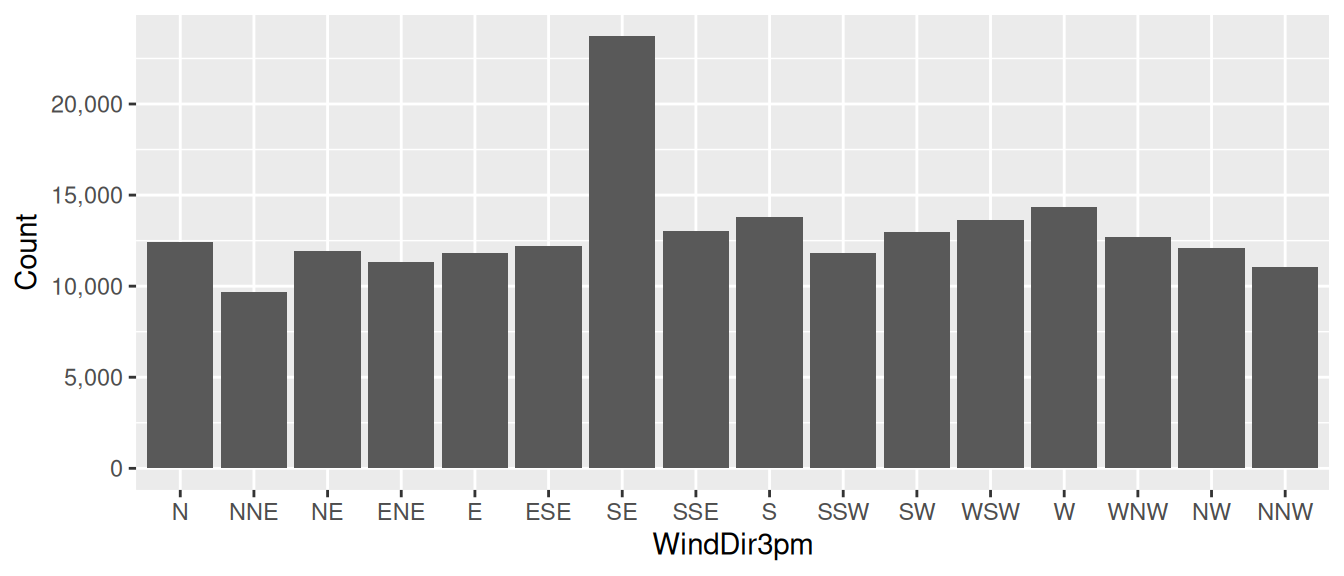## 11.4 Bar Chart Basic

20200427ds %>%
ggplot(aes(x=wind_dir_3pm)) +
geom_bar() +
scale_y_continuous(labels=comma) +
labs(x=vnames["wind_dir_3pm"], y="Count")

A common and simple plot is the bar chart which displays bars with a height that corresponds to the number of observations having that value of the variable displayed on the x-axis. We use ggplot2::geom_bar() to add a bar chart layer to a plot. Only an x-axis aesthetic is required using ggplot2::aes() with the x= option. The y-axis is automatically computed.

In our example we pipe the dataset on to ggplot2::ggplot(), specifying the x-axis as the categoric variable wind_dir_3pm (the x-axis). The count is automatically determined from the dataset by ggplot2::geom_bar() which then adds the layer of bars to the plot.

The y-ticks use commas with the ggplot2::scale_continuous() function using the labels=comma option. It is crucial that for large numbers commas separate the thousands, so that the reader is able to easily read the number. There can be catastrophic outcomes from a misreading of numbers.

The x-axis and y-axis labels are set using ggplot2::labs() with the x= and y= options. The x-label uses the original dataset’s variable name as recorded in the template variable vname (i.e., WindDir3pm). The y-label is set to be Count.

Your donation will support ongoing development and give you access to the PDF version of this book. Desktop Survival Guides include Data Science, GNU/Linux, and MLHub. Books available on Amazon include Data Mining with Rattle and Essentials of Data Science. Popular open source software includes rattle, wajig, and mlhub. Hosted by Togaware, a pioneer of free and open source software since 1984.